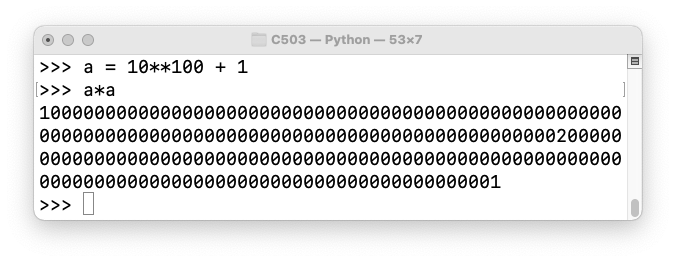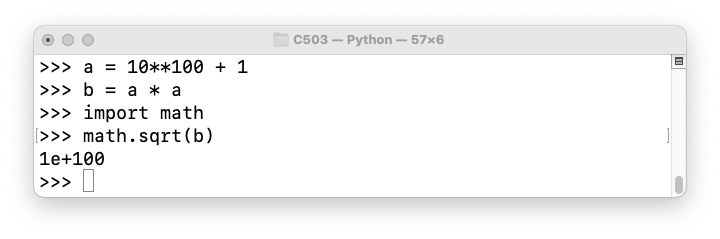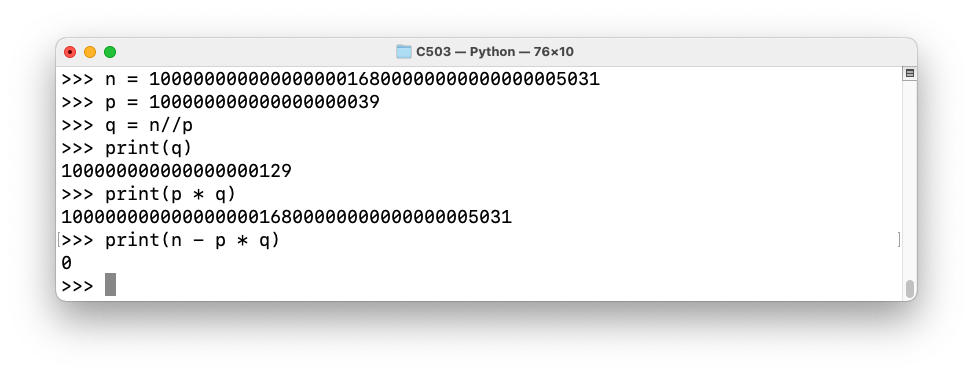C 504: Factoring Large Numbers (10 pts + 10 extra)

What you need:
• Any computer with Python 3.

Purpose

To factor large numbers which are the product of two primes that are close together.

Background

In general, factoring a large number is difficult or impossible, if the number is the product of two random large primes. However, if you have some information about the primes, it can be easy. In this case the weakess is that the two primes are close to one another.

1: Accurate Square Roots in Python

Multiplication in Python

Execute these commands at a Terminal or Command Prompt:
python3
a = 1001
a*a
As shown below, Python calculates the square of a correctly.Large Integers in Python

For simple operations like multiplication and division, Python handles large integers accurately by default. To see that, execute these commands at the Python prompt:
a = 10**100 + 1
a*a
As shown below, Python calculates the square of that 100-digit number correctly also.Testing math.sqrt()

However, the math.sqrt() function is not so precise. To see that, execute these commands at the Python prompt:
a = 10**100 + 1
b = a * a
import math
math.sqrt(b)
As shown below, math.sqrt() gets the wrong answer. Evidently it's rounding the numbers off.Using the Decimal Library

The Decimal library can be adjusted to be as precise as you like, solving this problem.

To see that, execute these commands at the Python prompt:

a = 10**100 + 1
b = a * a
from decimal import *
getcontext().prec = 200
Decimal(b).sqrt()
Now we get the right answer, as shown below.2. Example: Factoring a Large Number

Consider this question:

What are the factors of this number? Hint: there are two prime factors, and they are close to one another.

10000000000000000016800000000000000005031

Finding the Square Root

Since the two factors are close together, they must both be close to the square root of that number.

To find the square root, execute these commands at the Python prompt:

n = 10000000000000000016800000000000000005031
from decimal import *
getcontext().prec = 50
Decimal(n).sqrt()
The square root is 100000000000000000083 plus a fraction, as shown below.Testing Candidate Factors

One factor must be below the square root, and one above it. Also, since both factors are prime, they must both be odd.

So one way to find a factor is to test all odd numbers below the square root.

To do that, execute these commands at the Python prompt. Press Enter twice after the last command.

n = 10000000000000000016800000000000000005031
p0 = 100000000000000000083
for p in range(p0, p0-50, -2):
print(p, n%p)

As shown below, the modulus is zero when p is 100000000000000000039, so that's a factor.Finding q

To find the other factor, just divide n by p. To do that, and test the result, execute these commands at the Python prompt.

Notice the "//" operator in the third line: that performs "floor" division, returning an integer value. The "/" operator returns a floating point value, which is not what we want here.

n = 10000000000000000016800000000000000005031
p = 100000000000000000039
q = n//p
print(q)
print(p * q)
print(n - p * q)
As shown below, q is easily found and verified.The factors are:

p = 100000000000000000039
q = 100000000000000000129

As shown above, the square root of n is 100000000000000000083 (plus a fraction).

As expected, one factor is above the square root and the other is below it.

C 504.1: Factor this number (10 pts)

123459259296296790129629703704567911111222220989329646370537655992609296463211544461111289984805767

The flag is the smaller prime factor, as one long integer, like 11111111111111111111111111111111

C 504.2: Factor this number (10 pts extra)

2457319490775870034107936327697724401721210936487723795115696610653082228345978452724879092419462602801287921034412592451829320597304383170626854710604026609207557310932504074259543909051122202199219

The flag is the smaller prime factor, as one long integer, like 11111111111111111111111111111111

Posted 11-9-17 by Sam Bowne
Added to Crypto Hero 4-16-18 6:43 am
Ported to new scoring engine 7-8-19
Extra credit points specified 9-10-20
Updated for Python 3 11-10-21